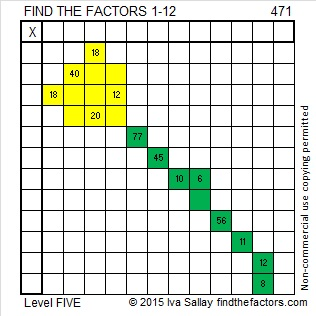# 471 and Level 5

471 is the sum of some consecutive prime numbers. One of my readers has listed those primes in the comments.Print the puzzles or type the solution on this excel file:  12 Factors 2015-04-20

—————————————————————————————————

• 471 is a composite number.
• Prime factorization: 471 = 3 x 157
• The exponents in the prime factorization are 1 and 1. Adding one to each and multiplying we get (1 + 1)(1 + 1) = 2 x 2 = 4. Therefore 471 has exactly 4 factors.
• Factors of 471: 1, 3, 157, 471
• Factor pairs: 471 = 1 x 471 or 3 x 471
• 471 has no square factors that allow its square root to be simplified. √471 ≈ 21.70253—————————————————————————————————## 2 thoughts on “471 and Level 5”

1.abyssbrain

This is a lot easier than the last one 🙂

151 + 157 + 163 = 471

•ivasallay

Some are easier than others, for sure. Thank you for finding this one and putting it in the comments.

This site uses Akismet to reduce spam. Learn how your comment data is processed.